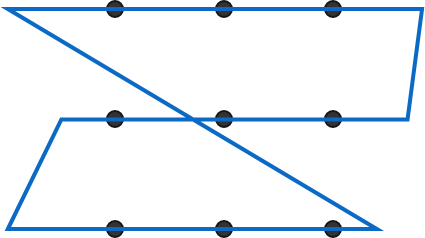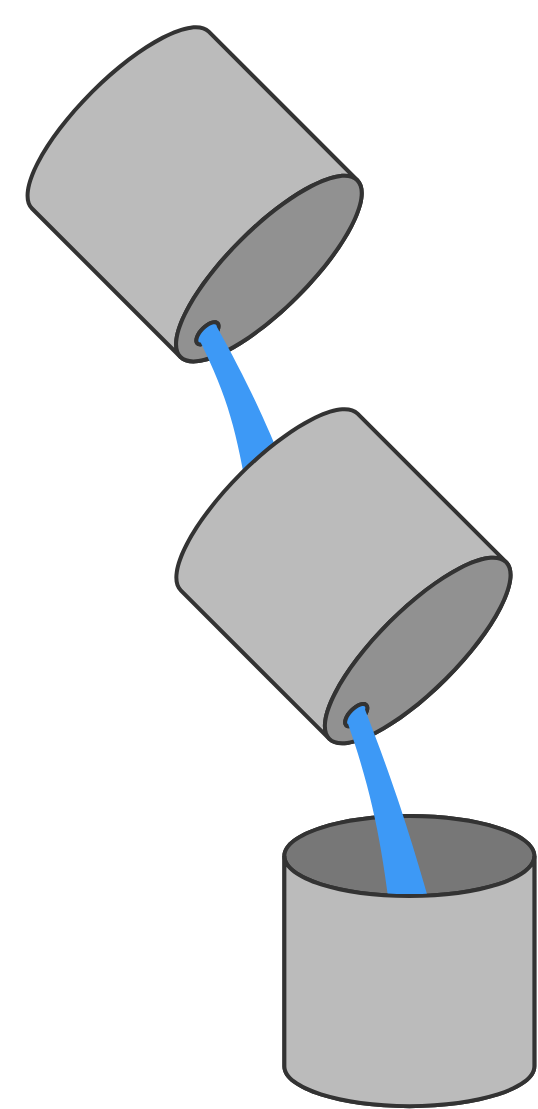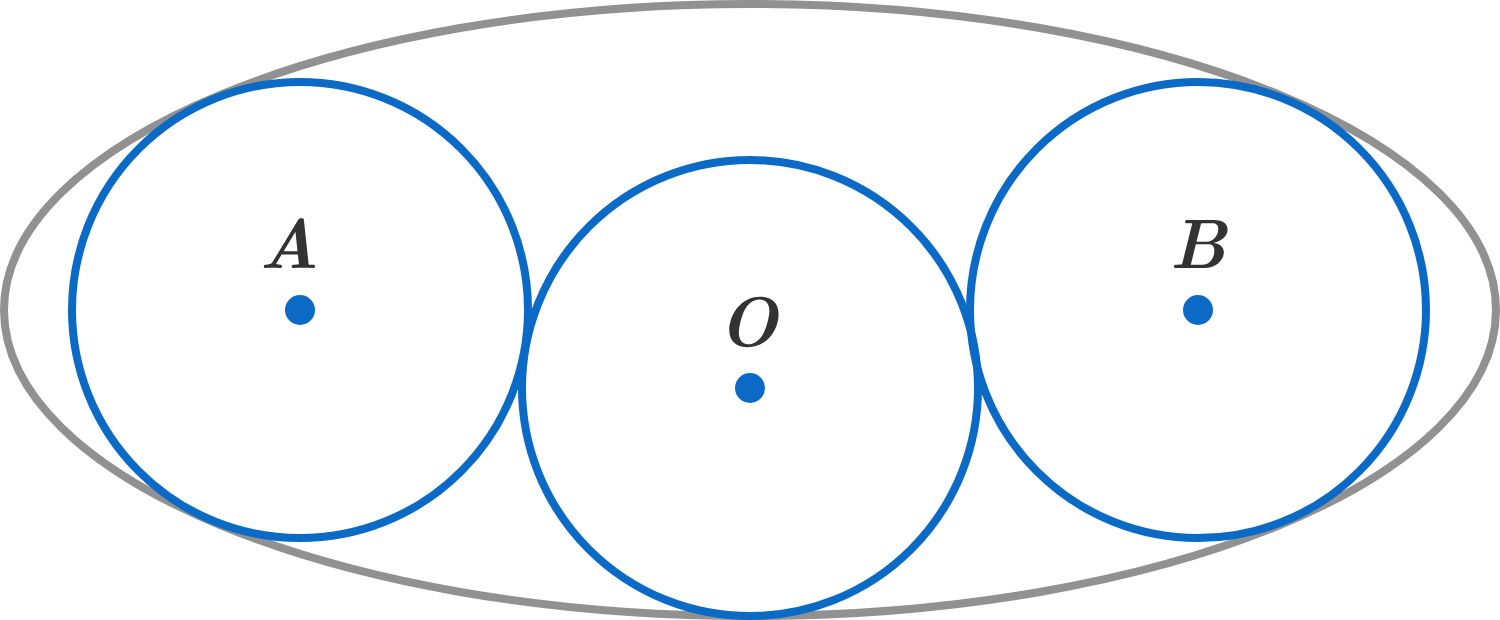# Problems of the Week

Contribute a problem

What is the minimum number of straight segments needed in a single closed loop, in order to pass through a $3\times 3$ square grid of points such that

• each point is intersected exactly once, and
• none of the lines have any grid point as their endpoint(s)?This closed loop meets the requirements, but can we do with fewer segments?

$\large a_0=a \quad \text{ and } \quad a_{n+1}=a^{a_n}$

What is the largest $a$ such that $a_n$ is convergent?There are three 50-gallon drums. Initially,

• Drum 1 contains 40 gallons of water with 3 pounds of dissolved salt,
• Drum 2 contains 10 gallons of water with 2 pounds of dissolved salt, and
• Drum 3 is empty.

While it still has water, Drum 1 empties into Drum 2 at a rate of 5 gallons per minute. While it still has water, Drum 2 empties into Drum 3 at a rate of 4 gallons per minute.

When Drum 2 reaches its maximum water level (though still not completely full), how many pounds of dissolved salt does it contain?

Assume that the salt water solutions are perfectly mixed at all times.Three identical circles are packed in an ellipse, as shown. Let $A,O,B$ be the centers of these circles (from left to right).

What is the angle $\angle AOB$ such that the area of the ellipse is minimized?

Whenever Richard takes a step, he chooses one of the four cardinal directions at random and moves one unit in that direction. For a certain number of steps $n,$ the probability of Richard starting at the origin and ending at the point $(4,4)$ is maximized.

What is $n?$

Note: If you decide to use a more brute force solution to this problem, it is highly recommended and encouraged that you use a calculator and/or a bit of programming.

×